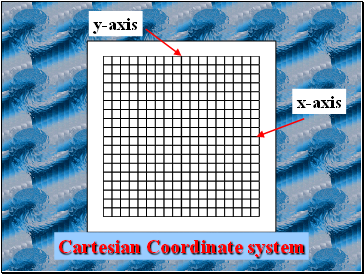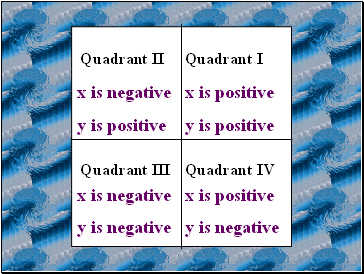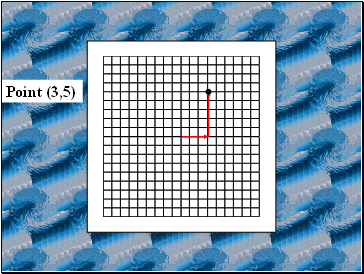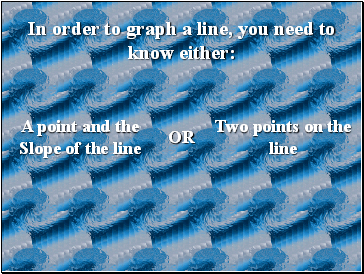# Graphing linesPage 1

#### WATCH ALL SLIDES

Slide 1## Graphing Linear Equations

Linear Equations can be graphed on a Cartesian Coordinate system

Slide 2x-axis

y-axis

Cartesian Coordinate system

Slide 3x-axis

y-axis

Origin

Slide 4All points on a graph are plotted by first finding the x-coordinate and then the y-coordinate

All points are written in the form ( x , y )

You always graph a point starting from the Origin

Slide 5x is positive

y is positive

x is negative

y is positive

x is negative

y is negative

x is positive

y is negative

Slide 6Point (3,5)

Slide 7In order to graph a line, you need to know either:

A point and the Slope of the line

Two points on the line

OR